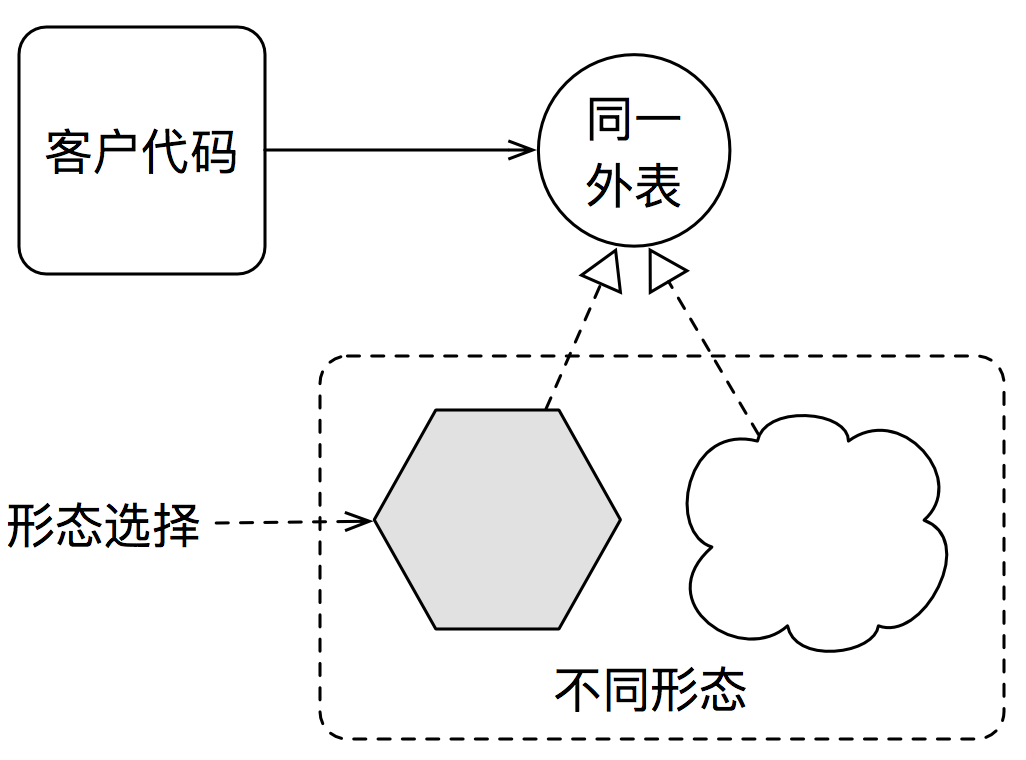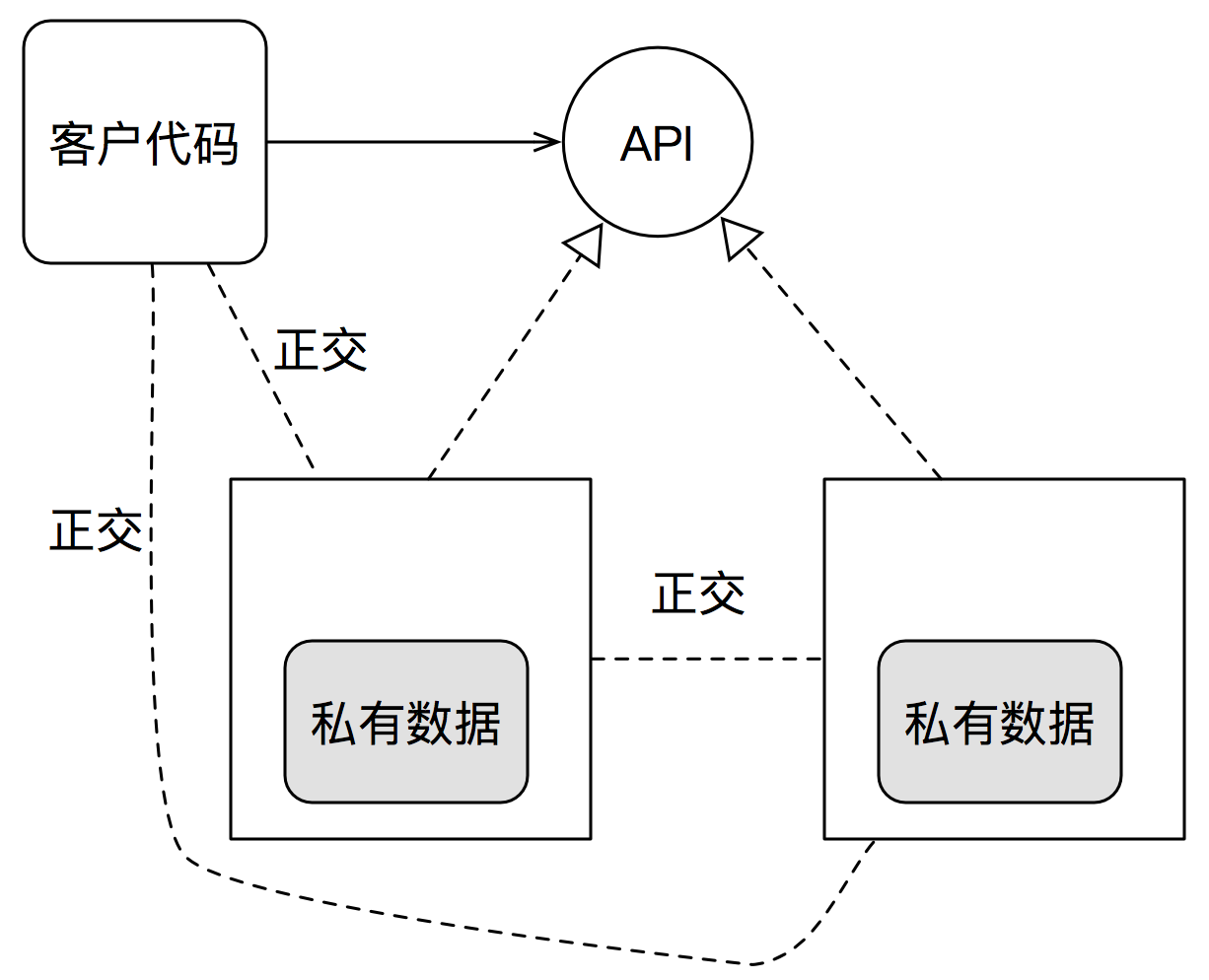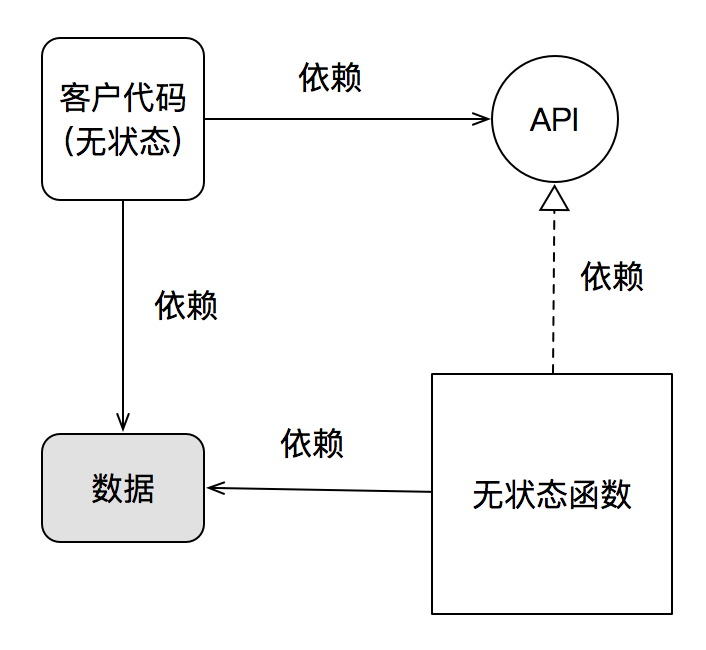@darwin-yuan 2016-06-30T03:33:42.000000Z 字数 8235 阅读 1690

# 多态，OO与FP

软件设计 OO FP

## 缘起

Erlang之父Joe Armstrong曾经写过一篇《Why OO Sucks》，被很多反OO的程序员——尤其是erlang社区的程序员——当作了大旗。

1. 数据结构和函数不应被绑在一起（Data structure and functions should not be bound together)；
2. 所有事物都不得不是对象(Everything has to be an object）；
3. 在面向对象语言里，数据类型定义散播在各处（In an OOPL data type definitions are spread out all over the place）;
4. 对象有私有状态（Objects have private state）。

## 一个例子

Joe在其著作《Programming Erlang》里，给出一个下面的例子：

area({rectangle, Width, Ht}) -> Width * Ht;area({circle, R})            -> 3.14159 * R * R;area({square, X})            -> X * X.

1. 数据结构和算法是分离的；
2. 没有私有状态，所有的数据结构信息都是公开的；
3. 对于不同类型数据的类似操作应该被放在一起。

enum ShapeType { Rectangle, Circle, Square }; struct Shape {    enum ShapeType kind;   union {         struct { int width, height; } rectangleData;     struct { int radius; } circleData;    struct { int side;   }  squareData  } shapeData; };double area(struct Shape* s) {   if( s->kind == Rectangle ) {     int width, ht;    width = s->shapeData.rectangleData.width;     ht = s->shapeData.rectangleData.ht;     return width * ht;   } else if ( s->kind == Circle ) {    // ...  } else if ( s->kind == Square ) {    // ...  }}

Joe认为这是一个与Erlang版本思想一致的C实现。都是使用模式匹配。差别只在于，Erlang的版本要比C版本要简练的多。

abstract class Shape {   abstract double area(); } class Circle extends Shape {  final double radius;  Circle(double radius) { this.radius = radius; }  double area() { return Math.PI * radius*radius; } } class Rectangle extends Shape {   final double ht;  final double width;  Rectangle(double width, double height) {     this.ht = height;    this.width = width;   }  double area() { return width * ht; } }class Square extends Shape {  final double side;  Square(double side) {     this.side = side;  }   double area() { return side * side; } } 

JoeOO的所有批评都是集中在现象级（包括他对OOPL的批评，错误的把某种OO语言的现象当做OOPL本身)，却没有深入到软件设计的挑战，目标和解决方案一级来探讨。这也是他写出那篇充满谬误的文章的最根本原因。

## 选择问题

1. 客户代码(用户)
2. 同一外表(抽象)
3. 不同形态(细节)
4. 形态选择(映射)1. 形态的变化，是一个选择问题，因而与其它选择形式，比如if-elseswitch-case，模式匹配等，可以等价转换；
2. 多态通过同一外表，封装了形态的变化；让客户代码不再受形态变化的影响，从而让系统具备更好的正交性。（多种不同形态间也是正交的）
3. 形态选择的过程，其实是一个组合的过程：把客户代码与某种具体形态进行组合。这是组合式设计的源泉之一。

## 多态与组合式设计

map :: (a -> b) -> [a] -> [b]
~

C程序员则会使用callback function的方式达到类似的效果。

Erlang由于是弱类型，所以并不存在参数化多态的问题。

C++程序员，可以将其看作范型编程中的模版。事实上，模版也是一种参数化多态

FP以参数化多态，以及朴素的运行时多态为基础，构成了FP自底向上的组合设计方法：通过将一个个可以适应类型变化的小函数逐级组合，最后得到更为强大的计算功能

## OO ROCKS!!!

Erlang作为一门FP语言，想必Joe一定是认可基于运行时多态的设计思想的。

1. OOclass为单位，可以定义一个接口集
2. 如果数据是一种实现细节，OO可以将数据进行信息隐藏。

struct Action{   virtual Status exec(TransactionInfo&) = 0;   virtual Status handleEvent(TransactionInfo&, const Event&) = 0;   virtual Status stop(TransactionInfo&) = 0;   virtual void kill(TransactionInfo&) = 0;   virtual ~Action() {}};## AD-HOC多态 vs. SUBTYPE多态

area({rectangle, Width, Ht}) -> Width * Ht;area({circle, R})            -> 3.14159 * R * R;area({square, X})            -> X * X.

perimeter({rectangle, Width, Ht}) -> 2 * (Width + Ht);perimeter({circle, R})            -> 2 * 3.14159 * R;perimeter({square, X})            -> 4 * X.

interface Shape {   // untouched code  double perimeter();} class Circle extends Shape {  // untouched code  double perimeter() { return Math.PI * 2 * radius; }} class Rectangle extends Shape {   // untouched code  double perimeter() { return (width + ht) * 2; } }class Square extends Shape {  // untouched code   double perimeter() { return 4 * side; }} 

Erlang需要做的修改是：

area({rectangle, Width, Ht}) -> Width * Ht;area({circle, R})            -> 3.14159 * R * R;area({square, X})            -> X * X;area({triangle, Data})       -> ... .perimeter({rectangle, Width, Ht}) -> 2 * (Width + Ht);perimeter({circle, R})            -> 2 * 3.14159 * R;perimeter({square, X})            -> 4 * X;perimeter({triangle, Data})       -> ... .

Java则无需改动任何原有代码，只需要增加一个新的类：

class Triangle extends Shape {  // Data  double area() { ... }  double perimeter() { ... }} 

1. 如果扩展一个操作，ad-hoc多态优于subtype多态
2. 如果扩展一个类型，subtype多态优于ad-hoc多态

area({rectangle, Width, Ht}) -> Width * Ht;area({circle, R})            -> 3.14159 * R * R;area({square, X})            -> X * X;area({triangle, Data1})       -> ... .perimeter({rectangle, Width, Ht}) -> 2 * (Width + Ht);perimeter({circle, R})            -> 2 * 3.14159 * R;perimeter({square, X})            -> 4 * X;perimeter({triangle, Data1})       -> ... .

Java版本则只需要修改一个类：

class Triangle extends Shape {  // Data1  double area() { ... }  double perimeter() { ... }} 

subtype多态再胜一局。

Erlang版本的设计，所有数据对于客户均是公开的，客户可以自由的访问它们。而一旦数据格式发生了变化，BANG!!!，所有相关的代码都需要修改。

## ADT 与 Existential Quantification

data Shape = Circle Float           | Rectangle Float Float           | Square Floatarea :: Shape -> Floatarea (Circle r)      = PI * r * rarea (Rectangle w h) = w * harea (Square s)      = s * sperimeter :: Shape -> Floatperimeter (Circle r)      = PI * r * 2perimeter (Rectangle w h) = (w + h) * 2perimeter (Square s)      = s * 4 

## 枚举 vs. subtype多态

Joe的例子，还给出了一个C语言的使用Union枚举的实现，这是一种典型的模拟ADT的实现方式（UnionADT Sum TypeC语言中的具体实现）。因而其带来的问题也和ADT一样。

## 结论

erlang确实有其非常出色的设计：比如其虚拟机提供的轻量级进程，以及Actor Model对于高并发，分布式，高可靠的支持，可复用库OTP对于快速构建通信类应用的支持等等都很不错。但所有这些都并不能构成OO Sucks的理由。

## 写在最后

Erlang has got all these things. It's got isolation, it's got polymorphism and it's got pure messaging. From that point of view, we might say it's the only object oriented language and perhaps I was a bit premature in saying that object oriented languages are about. You can try it and see it for yourself.
——Ralph Johnson, Joe Armstrong on the State of OOP• 私有
• 公开
• 删除